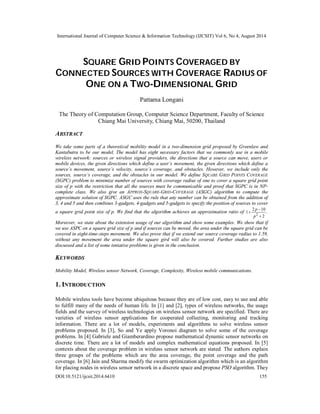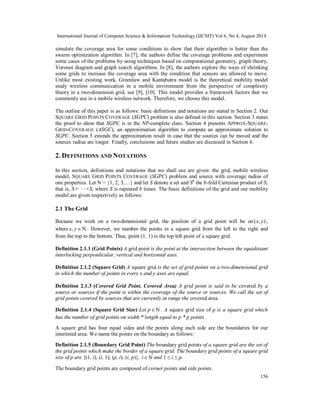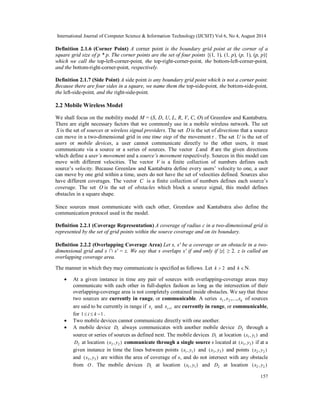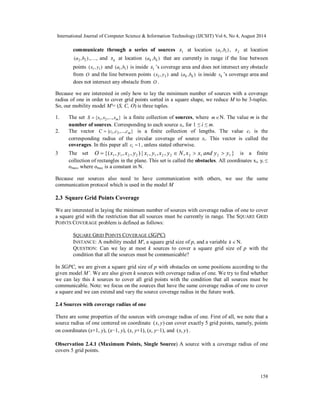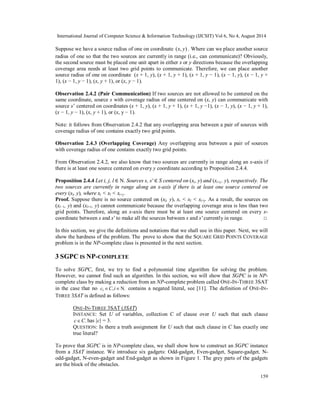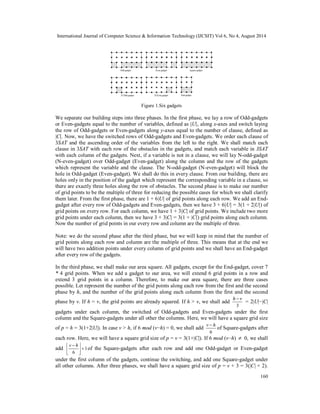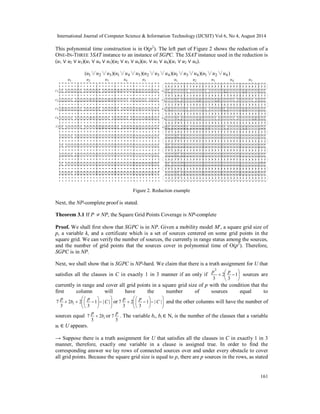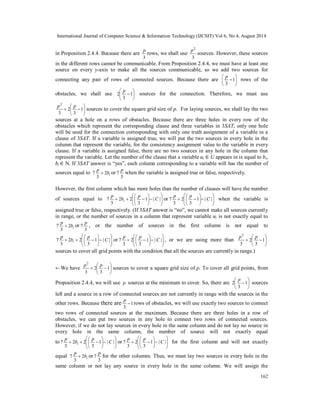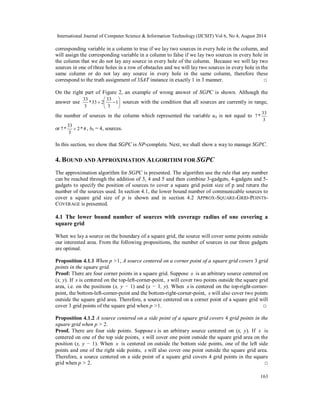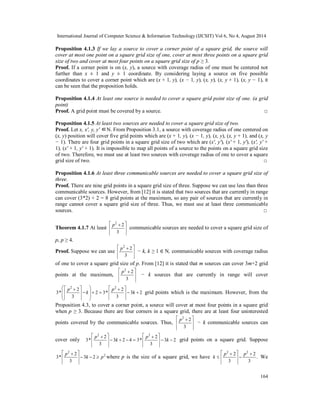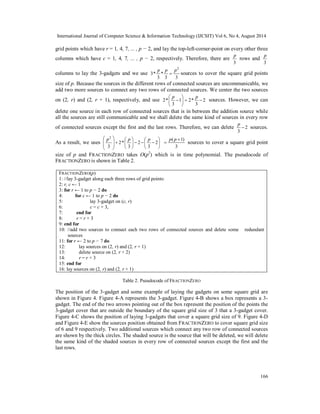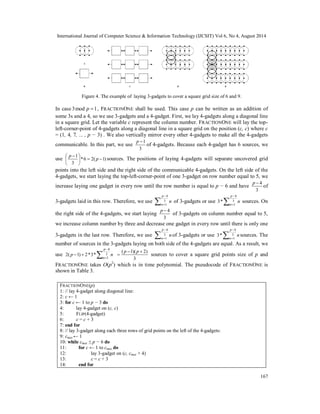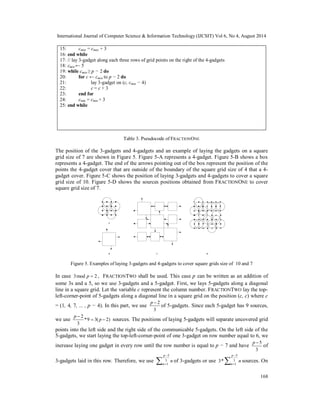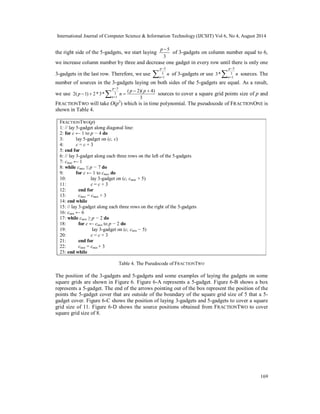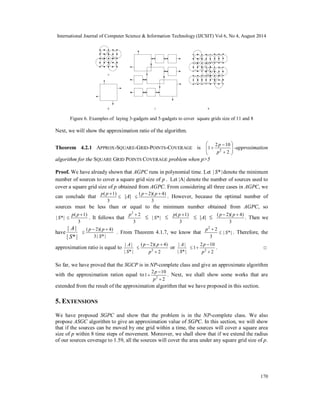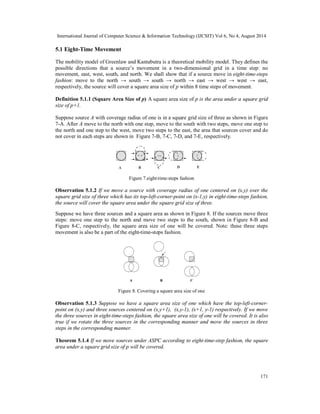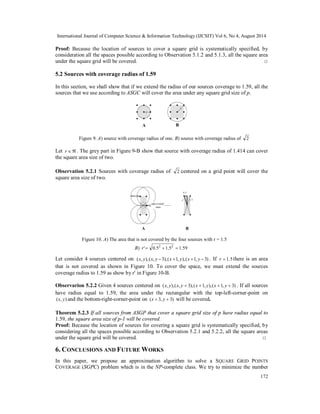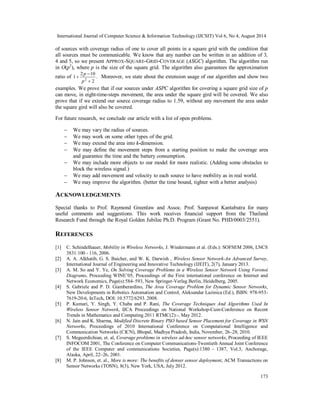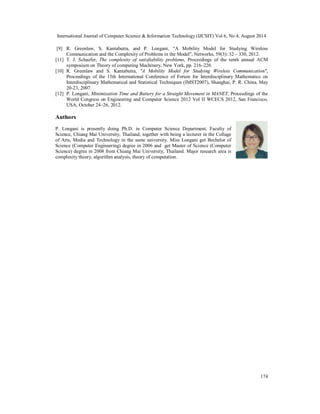Próximo SlideShare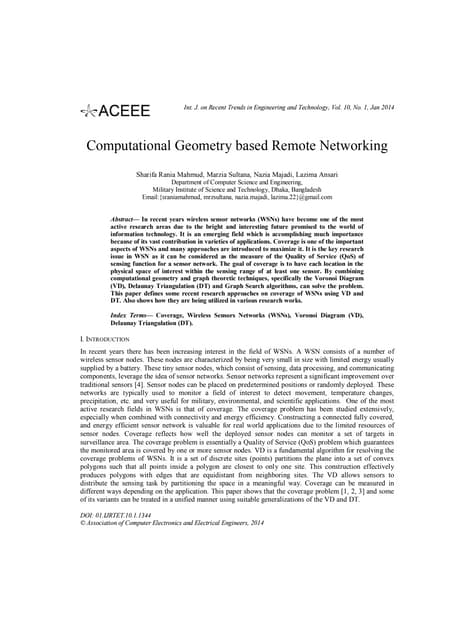Computational Geometry based Remote Networking
Cargando en ... 3
1 de 20

### Square grid points coveraged by

1. International Journal of Computer Science & Information Technology (IJCSIT) Vol 6, No 4, August 2014 SQUARE GRID POINTS COVERAGED BY CONNECTED SOURCES WITH COVERAGE RADIUS OF ONE ON A TWO-DIMENSIONAL GRID Pattama Longani The Theory of Computation Group, Computer Science Department, Faculty of Science Chiang Mai University, Chiang Mai, 50200, Thailand ABSTRACT We take some parts of a theoretical mobility model in a two-dimension grid proposed by Greenlaw and Kantabutra to be our model. The model has eight necessary factors that we commonly use in a mobile wireless network: sources or wireless signal providers, the directions that a source can move, users or mobile devices, the given directions which define a user’s movement, the given directions which define a source’s movement, source’s velocity, source’s coverage, and obstacles. However, we include only the sources, source’s coverage, and the obstacles in our model. We define SQUARE GRID POINTS COVERAGE (SGPC) problem to minimize number of sources with coverage radius of one to cover a square grid point size of p with the restriction that all the sources must be communicable and proof that SGPC is in NP-complete class. We also give an APPROX-SQUARE-GRID-COVERAGE (ASGC) algorithm to compute the approximate solution of SGPC. ASGC uses the rule that any number can be obtained from the addition of 3, 4 and 5 and then combines 3-gadgets, 4-gadgets and 5-gadgets to specify the position of sources to cover a square grid point size of p. We find that the algorithm achieves an approximation ratio of p . 1 2 10 2  2   p Moreover, we state about the extension usage of our algorithm and show some examples. We show that if we use ASPC on a square grid size of p and if sources can be moved, the area under the square grid can be covered in eight-time-steps movement. We also prove that if we extend our source coverage radius to 1.59, without any movement the area under the square gird will also be covered. Further studies are also discussed and a list of some tentative problems is given in the conclusion. KEYWORDS Mobility Model, Wireless sensor Network, Coverage, Complexity, Wireless mobile communications. 1. INTRODUCTION Mobile wireless tools have become ubiquitous because they are of low cost, easy to use and able to fulfill many of the needs of human life. In  and , types of wireless networks, the usage fields and the survey of wireless technologies on wireless sensor network are specified. There are varieties of wireless sensor applications for cooperated collecting, monitoring and tracking information. There are a lot of models, experiments and algorithms to solve wireless sensor problems proposed. In , So and Ye apply Voronoi diagram to solve some of the coverage problems. In  Gabriele and Giamberardino propose mathematical dynamic sensor networks on discrete time. There are a lot of models and complex mathematical equations proposed. In  contexts about the coverage problem in wireless sensor network are stated. The authors explain three groups of the problems which are the area coverage, the point coverage and the path coverage. In  Jain and Sharma modify the swarm optimization algorithm which is an algorithm for placing nodes in wireless sensor network in a discrete space and propose PSO algorithm. They DOI:10.5121/ijcsit.2014.6410 155
2. International Journal of Computer Science & Information Technology (IJCSIT) Vol 6, No 4, August 2014 simulate the coverage area for some conditions to show that their algorithm is better than the swarm optimization algorithm. In , the authors define the coverage problems and experiment some cases of the problems by using techniques based on computational geometry, graph theory, Voronoi diagram and graph search algorithms. In , the authors explore the ways of shrinking some grids to increase the coverage area with the condition that sensors are allowed to move. Unlike most existing work, Greenlaw and Kantabutra model is the theoretical mobility model study wireless communication in a mobile environment from the perspective of complexity theory in a two-dimension grid, see , . This model provides a framework factors that we commonly use in a mobile wireless network. Therefore, we choose this model. The outline of this paper is as follows: basic definitions and notations are stated in Section 2. Our SQUARE GRID POINTS COVERAGE (SGPC) problem is also defined in this section. Section 3 states the proof to show that SGPC is in the NP-complete class. Section 4 presents APPROX-SQUARE-GRID- COVERAGE (ASGC), an approximation algorithm to compute an approximate solution to SGPC. Section 5 extends the approximation result in case that the sources can be moved and the sources radius are longer. Finally, conclusions and future studies are discussed in Section 6. 2. DEFINITIONS AND NOTATIONS In this section, definitions and notations that we shall use are given: the grid, mobile wireless model, SQUARE GRID POINTS COVERAGE (SGPC) problem and source with coverage radius of one properties. Let N = {1, 2, 3,…} and let S denote a set and Sb the b-fold Cartesian product of S, that is, S×· · ·×S, where S is repeated b times. The basic definitions of the grid and our mobility model are given respectively as follows: 2.1 The Grid Because we work on a two-dimensional grid, the position of a grid point will be on (x, y) , where x, y . However, we number the points in a square grid from the left to the right and from the top to the bottom. Thus, point (1, 1) is the top left point of a square grid. Definition 2.1.1 (Grid Points) A grid point is the point at the intersection between the equidistant interlocking perpendicular, vertical and horizontal axes. Definition 2.1.2 (Square Grid) A square grid is the set of grid points on a two-dimensional grid in which the number of points in every x and y axes are equal. Definition 2.1.3 (Covered Grid Point, Covered Area) A grid point is said to be covered by a source or sources if the point is within the coverage of the source or sources. We call the set of grid points covered by sources that are currently in range the covered area. Definition 2.1.4 (Square Grid Size) Let p . A square grid size of p is a square grid which has the number of grid points on width * length equal to p * p points. A square grid has four equal sides and the points along each side are the boundaries for our interested area. We name the points on the boundary as follows: Definition 2.1.5 (Boundary Grid Point) The boundary grid points of a square grid are the set of the grid points which make the border of a square grid. The boundary grid points of a square grid size of p are {(1, i), (i, 1), (p, i), (i, p)}, i and 1 ≤ i ≤ p. The boundary grid points are composed of corner points and side points. 156
3. International Journal of Computer Science & Information Technology (IJCSIT) Vol 6, No 4, August 2014 Definition 2.1.6 (Corner Point) A corner point is the boundary grid point at the corner of a square grid size of p * p. The corner points are the set of four points {(1, 1), (1, p), (p, 1), (p, p)} which we call the top-left-corner-point, the top-right-corner-point, the bottom-left-corner-point, and the bottom-right-corner-point, respectively. Definition 2.1.7 (Side Point) A side point is any boundary grid point which is not a corner point. Because there are four sides in a square, we name them the top-side-point, the bottom-side-point, the left-side-point, and the right-side-point. 2.2 Mobile Wireless Model We shall focus on the mobility model M = (S, D, U, L, R, V, C, O) of Greenlaw and Kantabutra. There are eight necessary factors that we commonly use in a mobile wireless network. The set S is the set of sources or wireless signal providers. The set D is the set of directions that a source can move in a two-dimensional grid in one time step of the movement . The set U is the set of users or mobile devices, a user cannot communicate directly to the other users, it must communicate via a source or a series of sources. The vector L and R are the given directions which define a user’s movement and a source’s movement respectively. Sources in this model can move with different velocities. The vector V is a finite collection of numbers defines each source’s velocity. Because Greenlaw and Kantabutra define every users’ velocity to one, a user can move by one grid within a time, users do not have the set of velocities defined. Sources also have different coverages. The vector C is a finite collection of numbers defines each source’s coverage. The set O is the set of obstacles which block a source signal, this model defines obstacles in a square shape. Since sources must communicate with each other, Greenlaw and Kantabutra also define the communication protocol used in the model. Definition 2.2.1 (Coverage Representation) A coverage of radius c in a two-dimensional grid is represented by the set of grid points within the source coverage and on its boundary. Definition 2.2.2 (Overlapping Coverage Area) Let s, s′ be a coverage or an obstacle in a two-dimensional grid and s ∩ s′ = z. We say that s overlaps s′ if and only if |z| ≥ 2. z is called an 157 overlapping coverage area. The manner in which they may communicate is specified as follows. Let k  2 and k N.  At a given instance in time any pair of sources with overlapping-coverage areas may communicate with each other in full-duplex fashion as long as the intersection of their overlapping-coverage area is not completely contained inside obstacles. We say that these two sources are currently in range, or communicable. A series s1, s2 ,..., sk of sources are said to be currently in range if si and si1 are currently in range, or communicable, for 1  i  k 1 .  Two mobile devices cannot communicate directly with one another.  A mobile device D1 always communicates with another mobile device D2 through a source or series of sources as defined next. The mobile devices D1 at location (x1, y1) and D2 at location (x2 , y2 ) communicate through a single source s located at (x3, y3 ) if at a given instance in time the lines between points (x1, y1) and (x3, y3 ) and points (x2 , y2 ) and (x3, y3 ) are within the area of coverage of s, and do not intersect with any obstacle from O . The mobile devices D1 at location (x1, y1) and D2 at location (x2 , y2 )
4. International Journal of Computer Science & Information Technology (IJCSIT) Vol 6, No 4, August 2014 communicate through a series of sources s1 at location (a1,b1) , 2 s at location (a2,b2 ) ,…, and k s at location (ak ,bk ) that are currently in range if the line between points (x1, y1) and (a1,b1) is inside 1 s ’s coverage area and does not intersect any obstacle from O and the line between points (x2 , y2 ) and (ak ,bk ) is inside sk ’s coverage area and does not intersect any obstacle from O . Because we are interested in only how to lay the minimum number of sources with a coverage radius of one in order to cover grid points sorted in a square shape, we reduce M to be 3-tuples. So, our mobility model M′= (S, C, O) is three tuples. 1. The set S  {s1, s2,...,sm} is a finite collection of sources, where mN. The value m is the 158 number of sources. Corresponding to each source si, for 1 ≤ i ≤ m. 2. The vector C  {c1,c2,...,cm} is a finite collection of lengths. The value ci is the corresponding radius of the circular coverage of source si. This vector is called the coverages. In this paper all ci 1 , unless stated otherwise. 3 The set {( 1 , 1 , 2 , 2 ) | 1 , 1 , 2 , 2 , 2 1 2 1 } O  x y x y x y x y  N x  x and y  y is a finite collection of rectangles in the plane. This set is called the obstacles. All coordinates xi, yi ≤ omax, where omax is a constant in N. Because our sources also need to have communication with others, we use the same communication protocol which is used in the model M 2.3 Square Grid Points Coverage We are interested in laying the minimum number of sources with coverage radius of one to cover a square grid with the restriction that all sources must be currently in range. The SQUARE GRID POINTS COVERAGE problem is defined as follows: SQUARE GRID POINTS COVERAGE (SGPC) INSTANCE: A mobility model M′, a square grid size of p, and a variable k N. QUESTION: Can we lay at most k sources to cover a square grid size of p with the condition that all the sources must be communicable? In SGPC, we are given a square grid size of p with obstacles on some positions according to the given model M’. We are also given k sources with coverage radius of one. We try to find whether we can lay this k sources to cover all grid points with the condition that all sources must be communicable. Note: we focus on the sources that have the same coverage radius of one to cover a square and we can extend and vary the source coverage radius in the future work. 2.4 Sources with coverage radius of one There are some properties of the sources with coverage radius of one. First of all, we note that a source radius of one centered on coordinate (x, y) can cover exactly 5 grid points, namely, points on coordinates (x+1, y), (x−1, y), (x, y+1), (x, y−1), and (x, y) . Observation 2.4.1 (Maximum Points, Single Source) A source with a coverage radius of one covers 5 grid points.
7. International Journal of Computer Science & Information Technology (IJCSIT) Vol 6, No 4, August 2014 This polynomial time construction is in O(p2). The left part of Figure 2 shows the reduction of a ONE-IN-THREE 3SAT instance to an instance of SGPC. The 3SAT instance used in the reduction is (u1 ∨ u2 ∨ u3)(u1 ∨ u4 ∨ u5)(u2 ∨ u3 ∨ u4)(u1 ∨ u3 ∨ u4)(u1 ∨ u2 ∨ u4). p2 p sources are 7 p p C and the other columns will have the number of 7 p . The variable bi, bi ∈ N, is the number of the clauses that a variable 161 Figure 2. Reduction example  Next, the NP-complete proof is stated. Theorem 3.1 If P  NP, the Square Grid Points Coverage is NP-complete Proof. We shall first show that SGPC is in NP. Given a mobility model M, a square grid size of p, a variable k, and a certificate which is a set of sources centered on some grid points in the square grid. We can verify the number of sources, the currently in range status among the sources, and the number of grid points that the sources cover in polynomial time of O(p2). Therefore, SGPC is in NP. Next, we shall show that is SGPC is NP-hard. We claim that there is a truth assignment for U that satisfies all the clauses in C in exactly 1 in 3 manner if an only if   1   3 2 3 currently in range and cover all grid points in a square grid size of p with the condition that the first column will have the number of sources equal to     7 p b p C    1 | |       3 2 2 3     1  | | i or        3 2 3 sources equal p 2bi 7  or 3 3 ui ∈ U appears. → Suppose there is a truth assignment for U that satisfies all the clauses in C in exactly 1 in 3 manner, therefore, exactly one variable in a clause is assigned true. In order to find the corresponding answer we lay rows of connected sources over and under every obstacle to cover all grid points. Because the square grid size is equal to p, there are p sources in the rows, as stated
8. International Journal of Computer Science & Information Technology (IJCSIT) Vol 6, No 4, August 2014 p2 sources. However, these sources 2 p sources for the connection. Therefore, we must use p2 p sources to cover the square grid size of p. For laying sources, we shall lay the two sources at a hole on a rows of obstacles. Because there are three holes in every row of the obstacles which represent the corresponding clause and three variables in 3SAT, only one hole will be used for the connection corresponding with only one truth assignment of a variable in a clause of 3SAT. If a variable is assigned true, we will put the two sources in every hole in the column that represent the variable, for the consistency assignment value to the variable in every clause. If a variable is assigned false, there are no two sources in any hole in the column that represent the variable. Let the number of the clause that a variable ui ∈ U appears in is equal to bi, bi ∈ N. If 3SAT answer is “yes”, each column corresponding to a variable will has the number of sources equal to p 2bi 7 p p C when the variable is 7 p , or the number of sources in the first column is not equal to p2 p 7  p p C , or we are using more than   1 p2 p sources to cover a square grid size of p. To cover all grid points, from 2 p sources p  rows of obstacles, we will use exactly two sources to connect 7 p p C for the first column and will not exactly 7 p for the other columns. Thus, we must lay two sources in every hole in the 162 in Proposition 2.4.4. Because there are p rows, we shall use 3 3  in the different rows cannot be communicable. From Proposition 2.4.4, we must have at least one source on every y-axis to make all the sources communicable, so we add two sources for connecting any pair of rows of connected sources. Because there are  1  3  p rows of the  1 3  obstacles, we shall use       1  3 2 3 7  or 3 7 p when the variable is assigned true or false, respectively. 3   However, the first column which has more holes than the number of clauses will have the number of sources equal to  7 p  b   p  1 | C |       3 2 2 3     1  | | i or        3 2 3 assigned true or false, respectively. (If 3SAT answer is “no”, we cannot make all sources currently in range, or the number of sources in a column that represent variable ui is not exactly equal to bi p 2 3 7  or 3     7 p b p C    1 | |       3 2 2 3     1  | | i or        3 2 3   3 2 3 sources to cover all grid points with the condition that all the sources are currently in range.)   1  ←We have   3 2 3   1 3 Proposition 2.4.4, we will use p sources at the minimum to cover. So, there are   left and a source in a row of connected sources are not currently in range with the sources in the other rows. Because there are 1 3  two rows of  connected sources at the maximum. Because there are three holes in a row of obstacles, we can put two sources in any hole to connect two rows of connected sources. However, if we do not lay sources in every hole in the same column and do not lay no source in every hole in the same column, the number of source will not exactly equal to  7 p  b   p  1 | C |       3 2 2 3     1  | | i or        3 2 3 equal p 2bi 7  or 3 3 same column or not lay any source in every hole in the same column. We will assign the
9. International Journal of Computer Science & Information Technology (IJCSIT) Vol 6, No 4, August 2014  corresponding variable in a column to true if we lay two sources in every hole in the column, and will assign the corresponding variable in a column to false if we lay two sources in every hole in the column that we do not lay any source in every hole of the column. Because we will lay two sources in one of three holes in a row of obstacles and we will lay two sources in every hole in the same column or do not lay any source in every hole in the same column, therefore these correspond to the truth assignment of 3SAT instance in exactly 1 in 3 manner. □ On the right part of Figure 2, an example of wrong answer of SGPC is shown. Although the answer use 33 sources with the condition that all sources are currently in range, 7 * 33 163  *33 2 33 3   1  3 the number of sources in the column which represented the variable u4 is not equal to 3 7 * 33  , b4 = 4, sources. or 2 * 4 3 In this section, we show that SGPC is NP-complete. Next, we shall show a way to manage SGPC. 4. BOUND AND APPROXIMATION ALGORITHM FOR SGPC The approximation algorithm for SGPC is presented. The algorithm use the rule that any number can be reached through the addition of 3, 4 and 5 and then combine 3-gadgets, 4-gadgets and 5- gadgets to specify the position of sources to cover a square grid point size of p and return the number of the sources used. In section 4.1, the lower bound number of communicable sources to cover a square grid size of p is shown and in section 4.2 APPROX-SQUARE-GRID-POINTS-COVERAGE is presented. 4.1 The lower bound number of sources with coverage radius of one covering a square grid When we lay a source on the boundary of a square grid, the source will cover some points outside our interested area. From the following propositions, the number of sources in our three gadgets are optimal. Proposition 4.1.1 When p >1, A source centered on a corner point of a square grid covers 3 grid points in the square grid. Proof: There are four corner points in a square grid. Suppose s is an arbitrary source centered on (x, y). If s is centered on the top-left-corner-point, s will cover two points outside the square grid area, i.e. on the positions (x, y − 1) and (x − 1, y). When s is centered on the top-right-corner-point, the bottom-left-corner-point and the bottom-right-corner-point, s will also cover two points outside the square grid area. Therefore, a source centered on a corner point of a square grid will cover 3 grid points of the square grid when p >1. □ Proposition 4.1.2 A source centered on a side point of a square grid covers 4 grid points in the square grid when p > 2. Proof. There are four side points. Suppose s is an arbitrary source centered on (x, y). If s is centered on one of the top side points, s will cover one point outside the square grid area on the position (x, y − 1). When s is centered on outside the bottom side points, one of the left side points and one of the right side points, s will also cover one point outside the square grid area. Therefore, a source centered on a side point of a square grid covers 4 grid points in the square grid when p > 2. □
10. International Journal of Computer Science & Information Technology (IJCSIT) Vol 6, No 4, August 2014 Proposition 4.1.3 If we lay a source to cover a corner point of a square grid, the source will cover at most one point on a square grid size of one, cover at most three points on a square grid size of two and cover at most four points on a square grid size of p ≥ 3. Proof. If a corner point is on (x, y), a source with coverage radius of one must be centered not further than x ± 1 and y ± 1 coordinate. By considering laying a source on five possible coordinates to cover a corner point which are (x + 1, y), (x − 1, y), (x, y), (x, y + 1), (x, y − 1), it can be seen that the proposition holds. Proposition 4.1.4 At least one source is needed to cover a square grid point size of one. (a grid point) Proof. A grid point must be covered by a source. □ Proposition 4.1.5 At least two sources are needed to cover a square grid size of two. Proof. Let x, x′, y, y′ ∈ N. From Proposition 3.1, a source with coverage radius of one centered on (x, y) position will cover five grid points which are (x + 1, y), (x − 1, y), (x, y), (x, y + 1), and (x, y − 1). There are four grid points in a square grid size of two which are (x′, y′), (x′ + 1, y′), (x′, y′ + 1), (x′ + 1, y′ + 1). It is impossible to map all points of a source to the points on a square grid size of two. Therefore, we must use at least two sources with coverage radius of one to cover a square grid size of two. □ Proposition 4.1.6 At least three communicable sources are needed to cover a square grid size of three. Proof. There are nine grid points in a square grid size of three. Suppose we can use less than three communicable sources. However, from  it is stated that two sources that are currently in range can cover (3*2) + 2 = 8 grid points at the maximum, so any pair of sources that are currently in range cannot cover a square grid size of three. Thus, we must use at least three communicable sources. □ p2 2 communicable sources are needed to cover a square grid size of p2 2 − k, k ≥ 1 ∈ N, communicable sources with coverage radius p2 2 − k sources that are currently in range will cover   p k p k grid points which is the maximum. However, from the p2 2 − k communicable sources can   p k p k grid points on a square grid. Suppose k  p p . We 164 Theorem 4.1.7 At least        3 p, p ≥ 4. Proof. Suppose we can use        3 of one to cover a square grid size of p. From  it is stated that m sources can cover 3m+2 grid  points at the maximum,       3 3 2  2 2   2 3* 2 3  3* 2 3                      Proposition 4.3, to cover a corner point, a source will cover at most four points in a square grid when p ≥ 3. Because there are four corners in a square grid, there are at least four uninterested  points covered by the communicable sources. Thus,       3    2 2 3 2 4 3* 2 3* 2  cover only 3 2 3 3                   where p is the size of a square grid, we have 2 2  3* p 2  3 k  2  p 3      2 2 2  3 2 3     